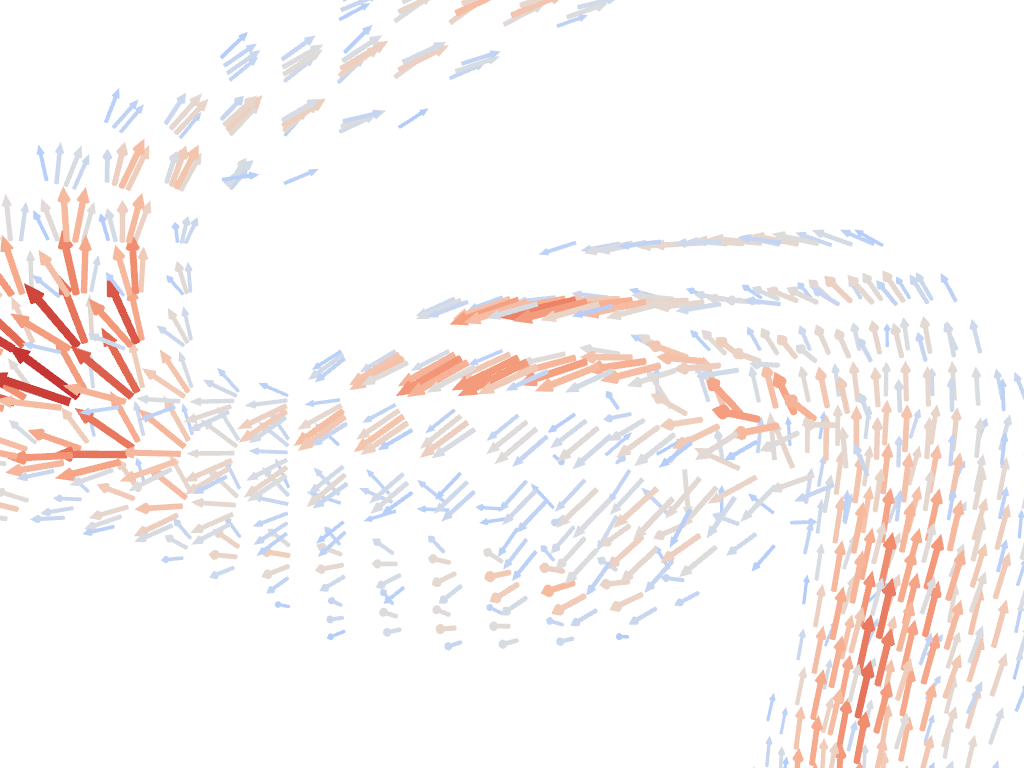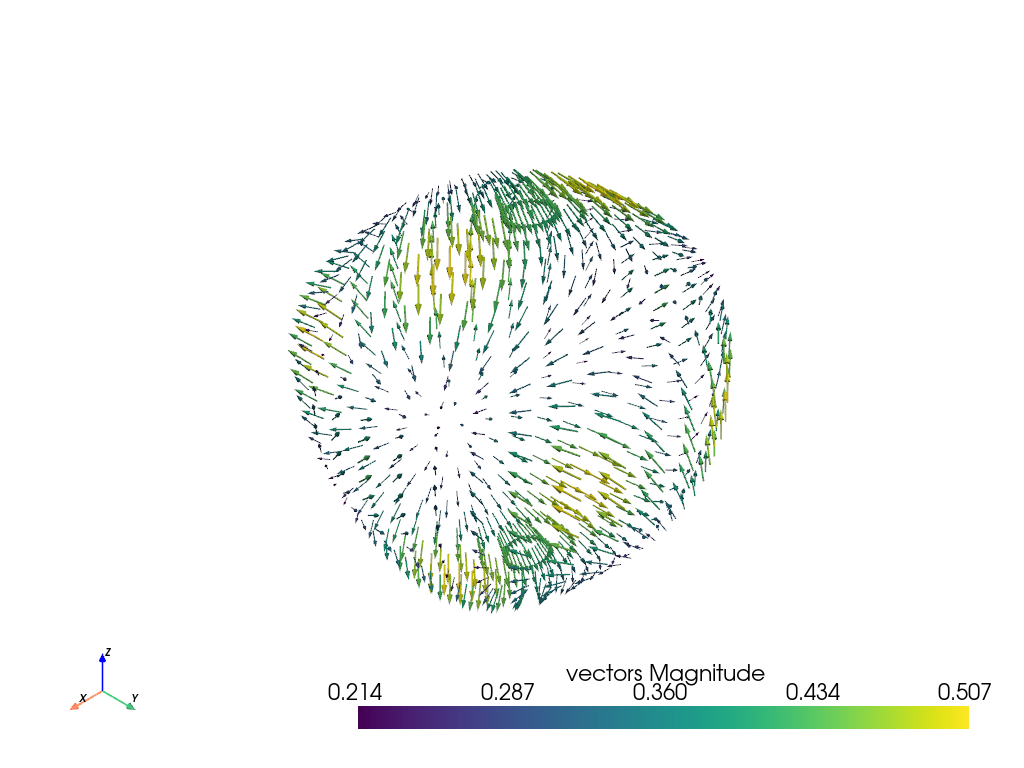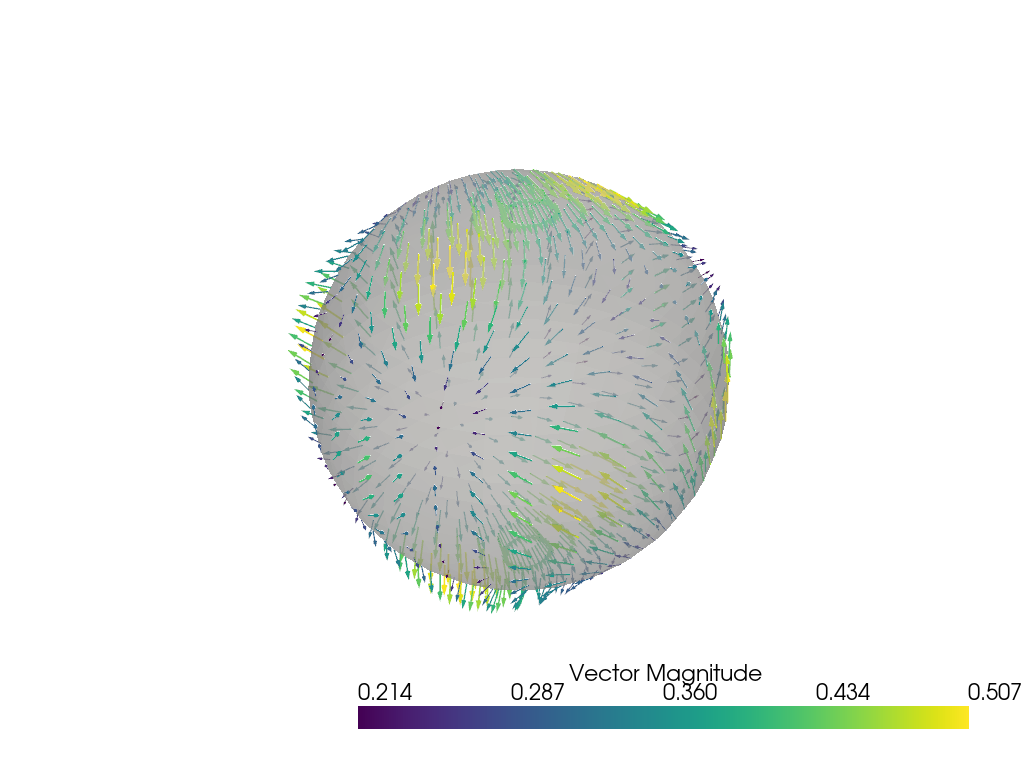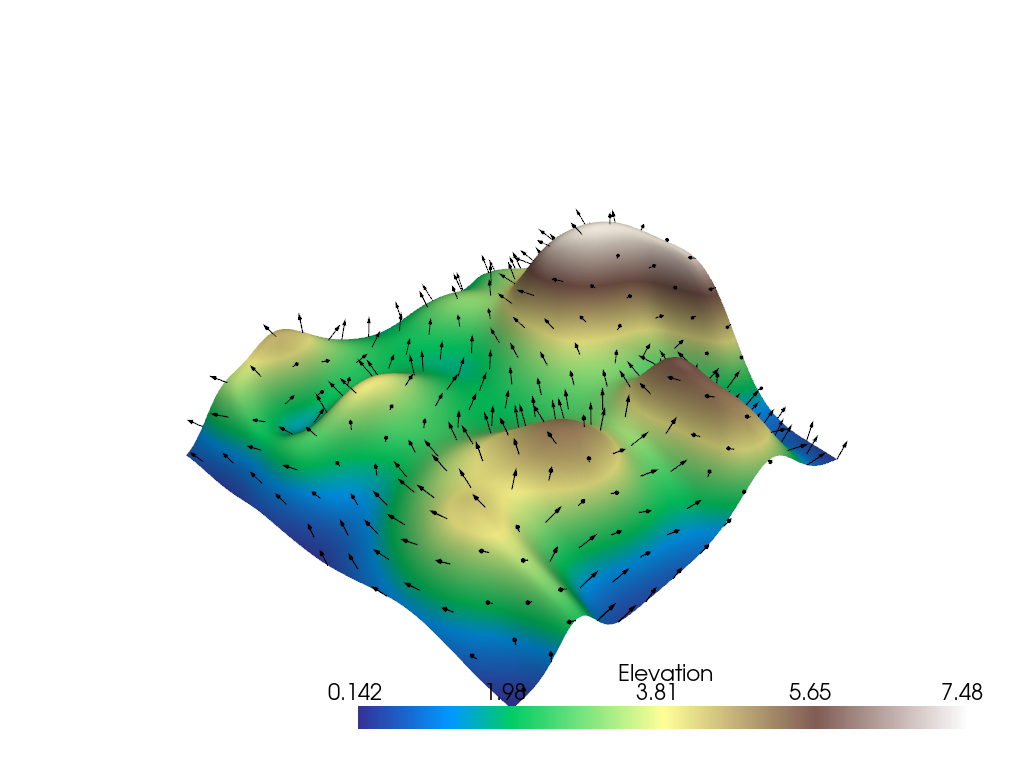# Plotting Glyphs (Vectors or PolyData)#

Use vectors in a dataset to plot and orient glyphs/geometric objects.

```import numpy as np

# sphinx_gallery_thumbnail_number = 4
import pyvista as pv
from pyvista import examples
```

Glyphying can be done via the `pyvista.DataSetFilters.glyph()` filter

```mesh = examples.download_carotid().threshold(145, scalars="scalars")
mesh["scalars"][mask] = 0  # null out smaller vectors

# Make a geometric object to use as the glyph
geom = pv.Arrow()  # This could be any dataset

# Perform the glyph
glyphs = mesh.glyph(orient="vectors", scale="scalars", factor=0.003, geom=geom)

# plot using the plotting class
pl = pv.Plotter()
pl.camera_position = [
(146.53, 91.28, 21.70),
(125.00, 94.45, 19.81),
(-0.086, 0.007, 0.996),
]  # view only part of the vector field
cpos = pl.show(return_cpos=True)
```Another approach is to load the vectors directly to the mesh object and then access the `pyvista.DataSet.arrows` property.

```sphere = pv.Sphere(radius=3.14)

# make cool swirly pattern
vectors = np.vstack(
(
np.sin(sphere.points[:, 0]),
np.cos(sphere.points[:, 1]),
np.cos(sphere.points[:, 2]),
)
).T

sphere["vectors"] = vectors * 0.3
sphere.set_active_vectors("vectors")

# plot just the arrows
sphere.arrows.plot()
```Plot the arrows and the sphere.

```p = pv.Plotter()
p.show()
```## Subset of Glyphs#

Sometimes you might not want glyphs for every node in the input dataset. In this case, you can choose to build glyphs for a subset of the input dataset by using a merging tolerance. Here we specify a merging tolerance of five percent which equates to five percent of the bounding box’s length.

```# Example dataset with normals

# create a subset of arrows using the glyph filter
arrows = mesh.glyph(scale="Normals", orient="Normals", tolerance=0.05)

p = pv.Plotter()